Baum-Welch AlgorithmNext: Gradient based method Up: Maximum Likelihood (ML) criterion Previous: Maximum Likelihood (ML) criterion

#### Baum-Welch Algorithm

This method can be derived using simple ``occurrence counting'' arguments or using calculus to maximize the auxiliary quantity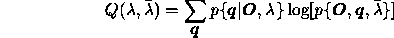over[],[, p 344-346,]. A special feature of the algorithm is the guaranteed convergence .
To describe the Baum-Welch algorithm, ( also known as Forward-Backward algorithm), we need to define two more auxiliary variables, in addition to the forward and backward variables defined in a previous section. These variables can however be expressed in terms of the forward and backward variables.

First one of those variables is defined as the probability of being in state i at t=t and in state j at t=t+1. Formally,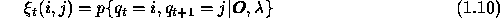This is the same as,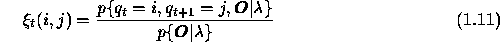Using forward and backward variables this can be expressed as,The second variable is the a posteriori probability,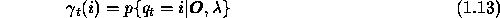that is the probability of being in state i at t=t, given the observation sequence and the model. In forward and backward variables this can be expressed by,One can see that the relationship between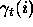andis given by,Now it is possible to describe the Baum-Welch learning process, where parameters of the HMM is updated in such a way to maximize the quantity,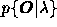. Assuming a starting model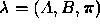,we calculate the '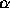's and ''s using the recursions 1.5 and 1.2, and then '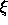's and '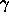's using 1.12 and 1.15. Next step is to update the HMM parameters according to eqns 1.16 to 1.18, known as re-estimation formulas.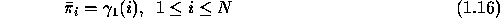These reestimation formulas can easily be modified to deal with the continuous density case too.